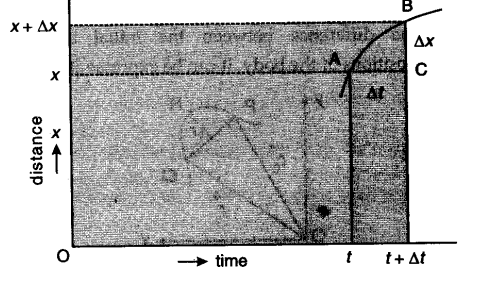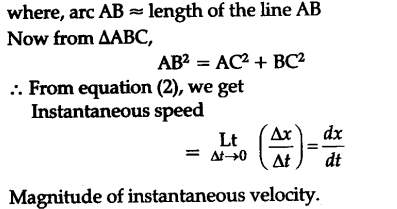# We have carefully distinguished between average speed and magnitude of average velocity

We have carefully distinguished between average speed and magnitude of average velocity. No such distinction is necessary when we consider instantaneous speed and magnitude of velocity. The instantaneous speed is always equal to the magnitude of instantaneous velocity. Why ?

The instantaneous velocity is defined as the velocity of an object at a particular instant of time.
The instantaneous speed is defined as the limiting value of the average speed. So, when time interval is very small, the magnitude of the displacement is effectively equal to the distance travelled by the object in the same small interval of time. Hence, both instantaneous velocity and instantaneous speed are equal in this case. This can be understood from the following as :